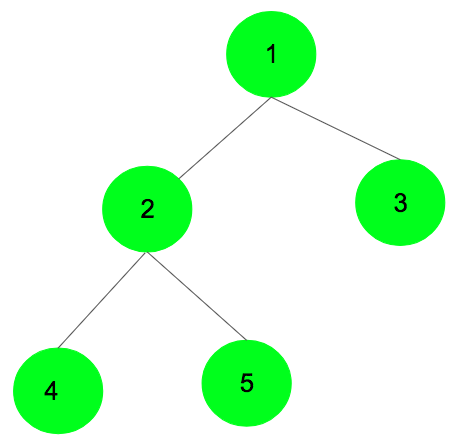# Deleting a binary tree using the delete keyword

• Difficulty Level : Easy
• Last Updated : 26 Jul, 2018

A recursive and a non-recursive program to delete an entire binary tree has already been discussed in the previous posts. In this post, deleting the entire binary tree using the delete keyword in C++ is discussed.

Declare a destructor function in the ‘BinaryTreeNode’ class which has been defined to create a tree node. Using ‘delete’ keyword on an object of a class deletes the entire binary tree., it’s destructor is called within the destructor. Use the ‘delete’ keyword for the children; so the destructors for the children will be called one by one, and the process will go on recursively until the entire binary tree is deleted. Consider the tree shown below, as soon as the destructor is called for the root i.e., ‘1’, it will call the destructors for ‘2’ and ‘3’, and 2 will then call the same for its left and right child with data ‘4’ and ‘5’ respectively. Eventually, the tree will be deleted in the order: 4->5->2->3->1 (Post-order)## Recommended: Please try your approach on {IDE} first, before moving on to the solution.

Below is the C++ implementation of the above approach:

 `// C++ program to delete the entire binary ` `// tree using the delete keyword ` `#include ` `using` `namespace` `std; ` ` `  `class` `BinaryTreeNode { ` ` `  `    ``// Making data members public to ` `    ``// avoid the usage of getter and setter functions ` `public``: ` `    ``int` `data; ` `    ``BinaryTreeNode* left; ` `    ``BinaryTreeNode* right; ` ` `  `    ``// Constructor function to ` `    ``// assign data to the node ` `    ``BinaryTreeNode(``int` `data) ` `    ``{ ` `        ``this``->data = data; ` `        ``this``->left = NULL; ` `        ``this``->right = NULL; ` `    ``} ` ` `  `    ``// Destructor function to delete the tree ` `    ``~BinaryTreeNode() ` `    ``{ ` `        ``// using keyword to delete the tree ` `        ``delete` `left; ` `        ``delete` `right; ` ` `  `        ``// printing the node which has been deleted ` `        ``cout << ``"Deleting "` `<< ``this``->data << endl; ` `    ``} ` `}; ` ` `  `// Driver Code ` `int` `main() ` `{ ` `    ``// Creating the nodes dynamically ` `    ``BinaryTreeNode* root = ``new` `BinaryTreeNode(1); ` `    ``BinaryTreeNode* node1 = ``new` `BinaryTreeNode(2); ` `    ``BinaryTreeNode* node2 = ``new` `BinaryTreeNode(3); ` `    ``BinaryTreeNode* node3 = ``new` `BinaryTreeNode(4); ` `    ``BinaryTreeNode* node4 = ``new` `BinaryTreeNode(5); ` ` `  `    ``// Creating the binary tree ` `    ``root->left = node1; ` `    ``root->right = node2; ` `    ``node1->left = node3; ` `    ``node1->right = node4; ` ` `  `    ``// Calls the destructor function which actually deletes the tree entirely ` `    ``delete` `root; ` ` `  `    ``return` `0; ` `}`

Output:

```Deleting 4
Deleting 5
Deleting 2
Deleting 3
Deleting 1
```

My Personal Notes arrow_drop_up
Recommended Articles
Page :